edHelper subscribers - Create a new printable

Sample edHelper.com - Algebra Worksheet

 Name _____________________________Date ___________________
The Law of Cosines
Use the law of cosines to find all of the unknown sides and angles in each triangle.

1.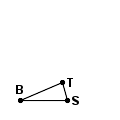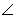S = 74BS
=  37.6ST
=  15.2
2.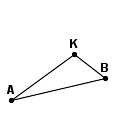AK
=  117AB
=  145.5BK
=  58.5
3.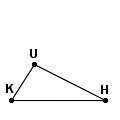KU
=  79.9KH
=  174.6HU
=  148.6
4.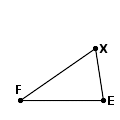FX
=  18.2FE
=  16.6EX
=  10.6
5.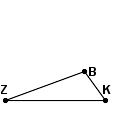B = 106ZB
=  168KB
=  72
6.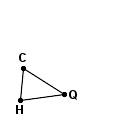Q = 41HQ
=  58.7QC
=  65.3
7.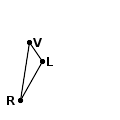RV
=  118RL
=  90LV
=  46
8.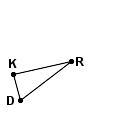K = 88DK
=  13.5RK
=  29.5
9.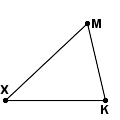XM
=  84XK
=  75KM
=  59.3

Sample
This is only a sample worksheet.

edHelper subscribers - Create a new printable